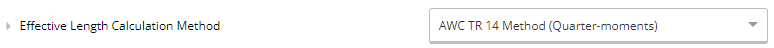# Generalized Lateral Stability Calculation for Wood Beams

To account for lateral stability in bending, the Canadian CSA O86 standard makes use of a factor $K_L$ which reduces the capacity of a member based on its length and different loading conditions. To account for different loading conditions, a table is provided that matches often-seen conditions with effective lengths. For instance, a uniformly loaded beam will have an effective length of $1.92L$, whereas a centre-loaded simply supported beam will have an effective length of $1.61L$ if there are no intermediate supports. However, these loading conditions are highly idealized and often do not align with real-world conditions, leaving designers wondering what effective length to use. For instance, what if a girder supports a uniformly distributed load but also a point load from a column? The code provides a catch-all effective length of $1.92L$, however this can often be excessively conservative.

Looking at Canada's neighbours to the south, the United States also makes use of essentially the same effective length formulas in the American Wood Council (AWC)'s National Design Specification (NDS). However, the AWC also provides an alternative design solution through its Technical Report 14 (TR 14). The report provides a completely different approach, based on directly calculating the critical buckling moment based on the loading conditions, instead of using an effective length. This is essentially the same method as what's used in calculating lateral-torsional buckling capacity in steel members - using the moments at quarter-points to calculate a $C_b$ factor, analogous to $\omega_2$ in the CSA S16 steel design standard. The advantage with this is that the quarter-moment method can be used with any loading conditions and doesn't require as much interpretation from the designer - which is perfect for implementation in a platform like ClearCalcs.

Indeed, that's what we offer you in ClearCalcs -  you can choose to use the TR 14 method in any wood beam or column design calculator by selecting it here:### Implementation of the TR14 Method with CSA O86

Although TR 14 provides a versatile solution to calculate lateral stability in wood beams, it can't quite be directly implemented with the CSA O86 standard. That's because beyond calculating the critical buckling moment, the report also provides a direct implementation in the NDS's formulas for inelastic buckling, which do differ from the CSA ones, which are based on the effective length method. So to use TR 14, we need to be a bit creative and find a way to convert the TR 14 buckling moment to an effective length that's useable in the CSA standard. This is what we'll describe next:

Since TR 14 is entirely based on finding the critical buckling moment, let's start with that calculation. For rectangular members, the report provides a simple formula (Eq. 18) that can be used:

$M_{cr} = \frac{1.3 C_b C_e E'_{y05} I_y}{\ell_u}$

where $C_b$ is the moment modification factor and $C_e$ is an adjustment if the load is applied above the centre of the beam - you can see their derivations and calculations in the TR 14 document. $E'_{y05}$ corresponds to the "design" modulus of elasticity in the weak axis, $I_y$ to the weak-axis moment of inertia, and $\ell_u$ to the unbraced length.

Looking at the NDS (Eq. 3.3-6) and TR14 (Eq. 15) next to each other, we can see that the final value for $C_L$ (equivalent to $K_L$ in Canada) is calculated the same way in both publications:

$C_L = \frac{1+\alpha_b}{1.90} - \sqrt{ \left(\frac{1+\alpha_b}{1.90}\right)^2 - \frac{\alpha_b}{0.95} }$

where $\alpha_b$ is taken as $F_{bE}/F_b^{*}$ in the NDS and as  $M_{cr} / M^{*}$ in TR14. The "$*$" superscript corresponds to the strength calculated considering every adjustment factor other than $C_L$, so we can say that $M^{*} = S F_b^{*}$ where $S$ is the section modulus. So we can say that:

$\frac{M_{cr}}{M^{*}} = \frac{F_{bE}}{F_b^*}$

which can be further simplified:

$F_{bE} = M_{cr}/S$

That makes sense - $F_{bE}$ corresponds to the elastic buckling stress. This formula shows exactly how the "connection" is made between TR 14 and the NDS! Now to bring it to Canada... Let's look at how $F_{bE}$ is calculated in the NDS (Eq. 3.3-6):

$F_{bE} = \frac{1.20 E_{min}'}{R_B^2}$

Note that $E_{min}'$ is the same as $E_{y05}'$. If we look at $R_B$ next, we find this definition (Eq. 3.3-5):

$R_B = \sqrt{\frac{\ell_e d}{b^2}}$

Where $\ell_e$ is the effective length, $d$ and $b$ are the depth and width, respectively. Look familiar? That's because it's exactly the same equation as we use for $C_B$ in CSA O86 (Cl 7.5.6.4.3):

$C_B = \sqrt{\frac{L_e d}{b^2}} = R_B$

Our first connection between the standards! Let's go back now and combine a few equations we looked at. Combining the two equations we found for $F_{bE}$, we can arrive to this:

$C_B^2 = R_B^2 = \frac{1.2 E'_{min} S}{M_{cr}}$

Adding in the formula for $M_{cr}$ now and simplifiying:

$C_B^2 = \frac{1.2 S \ell_u}{1.3 C_b C_e I_y}$

From basic formulae, we know that $I_y = db^3/12$ and $S = bd^2/6$. So we further simplify:

$C_B^2 = \frac{d}{b^2} \times 1.846 \frac{\ell_u}{C_b C_e}$

Looking at the equation for $C_B$ again, it's clear that the right term here is our effective length! So we can thus conclude that our effective length can be taken as such:

$L_e = 1.846 \ell_u / C_bC_e$

This is the formula we use in ClearCalcs to calculate the effective length for the lateral stability factor. With this method, we're able to consider and provide accurate results for any loading condition automatically.